﻿ The Power of Names# The Power of Names

Spread is the go-to control for embedding Microsoft Excel-compatible spreadsheet functionality into your .NET, ASP.NET, Silverlight, WPF, Windows Store, and COM applications. For all platforms, the control is easy to customize using the Spread Designer (or code if you prefer). In this example we'll show how to modify your spreadsheet by assigning names.

Most people familiar with spreadsheets know about assigning names for cells and ranges. The "name box" shows the name for the cell or range selected in Spread and Excel: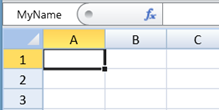MyName set for cell A1

Names can be much more though; for example, any valid formula expression can be set for a name. The following simple example demonstrates this: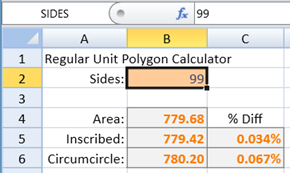Example 1: Polygon, Inscribed, and Circumscribed Circle Area Calculator

Spread for WPF includes a Spread Designer, which I used to create a simple calculator. It accepts one input value, the number of sides for a regular unit polygon, and computes some values from it. The calculation uses the name "SIDES" to get the value the user types in cell B2, and each of the formulas in the gray cells directly references one of the following names to do the calculation: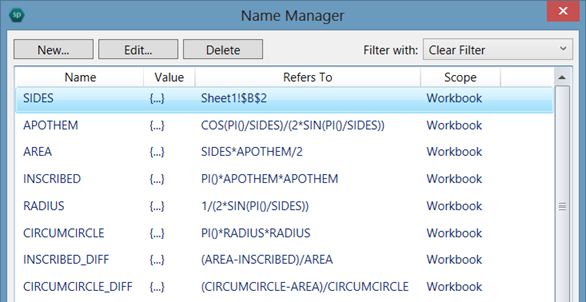The formulas use simple trigonometry functions to calculate the areas, and each step in the calculations is easier to read and follow because of the names used. Using names for your formulas also lets you use the Name Manager to view all your formulas at once, which can make debugging easier. You can find the Name Manager in the Formulas tab within the Spread Designer's ribbon bar: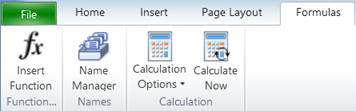Name Manager is in the Formulas tab in the Spread Designer ribbon bar

Since the scope of the names is "Workbook," we can reuse them in another sheet to do the same calculation, but this time instead of just performing the calculation once, we can make a table that shows the same calculation in each row, and compute the values based on a value for "SIDES" in that row. To do that, we define the name "SIDES" locally in the sheet to use a relative reference. For this example, the table will start in row 3 and the value for "SIDES" will be in column A, so the relative reference for "SIDES" in Sheet2 needs to be "\$A3". It is important to go to that sheet and cell first (i.e., make Sheet2 the active sheet and cell A3 the active cell), before adding the local name: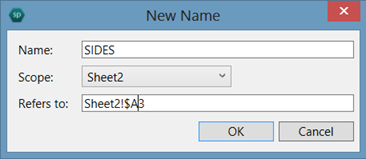Local definition of SIDES scoped to Sheet2

By default, Spread will fill in the New Name dialog's "Refers to:" field with a reference to the current active cell, which in this case is "Sheet2!\$A\$3". Using a relative reference for the row (i.e., removing the '\$' before the '3' as shown above) means that the cell referred to by "SIDES" will be computed relative to the cell containing the formula (i.e., formulas in row 3 will get the value from A3, formulas in row 4 will get the value from A4, and so on). Now that we have defined the name, we can add the values in the cells. You can quickly fill the cells with drag fill. Simply type the first few values 3, 4, 5, in cells A3, A4, and A5, then select those cells and drag the fill cursor from the bottom right of the cell: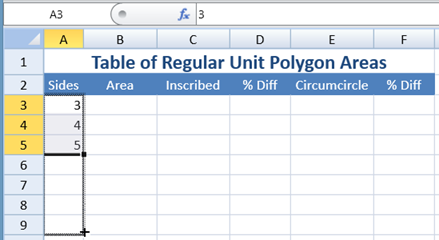Use drag-fill to fill the series values for SIDES

Then to complete the table, we can type the formulas into cells B3, C3, and so on, to compute the values just like in Example 1, but this time the value for "SIDES" will come from column A: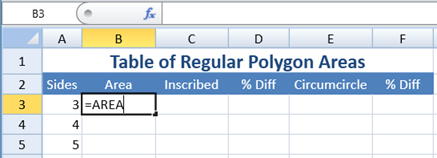Enter Formulas in row 3

Once the formulas are entered, we can format the values as numbers and percentages with three decimal places, using the ribbon bar controls in the Home tab: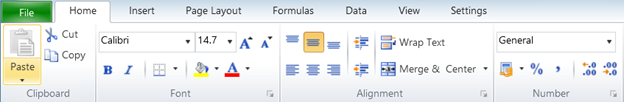Use the buttons in the Number section to set percent format and change decimal places

To format the values:

1. Select cell D3, press CTRL, and click cell F3 to select it too.
2. Then click the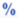button in the ribbon bar Number section to change the cell format to percentage.
3. Next, click the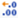button to the right of it to increase the decimal places until it shows 3 decimal places.
4. Select cell B3, press CTRL, and click cell C3 and E3 to select those cells too.
5. Then click the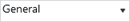cell format drop down and select Number format.
6. Next, click thebutton to increase the decimal places to 3.

The result should look like this: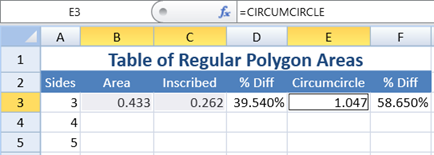Formatting cells is quick and easy with Spread Designer

Now we can select the cells B3:F3 and use drag-fill to complete the table: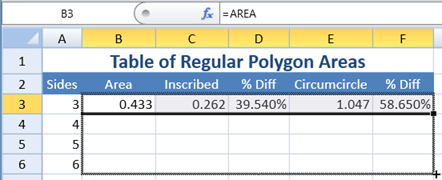Use drag-fill to copy formulas and formatting down

We end up with the completed table that looks like this: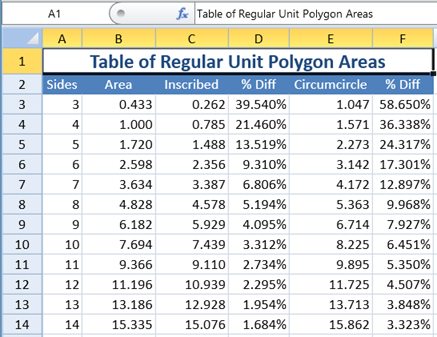Example 2: Table of Polygon Areas

# Getting Names to Work in Excel

Microsoft Excel does not work quite the same way as Spread, and the above example will not work in Excel. If we use the Spread Designer to save the above example workbook to XLSX and open it in Excel 2013, it looks like this: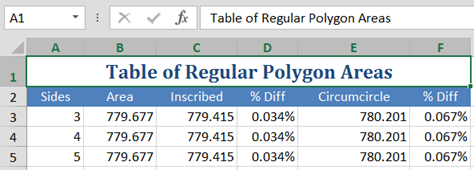Polygon Table in Excel 2013 doesn't work

The reason it works differently is because of the way that Excel evaluates name references in formulas. Since the names referenced in the cell formulas are defined with Workbook scope, Excel evaluates the references to "SIDES" in the formulas for those names using the global Workbook definition instead of the local definition of "SIDES" for Sheet2, so each row calculates the values using the same value in the cell Sheet1!B2. To make this table work when exported to XLSX and opened in Excel, we must define each name locally and explicitly reference the local Sheet2 definition of each name in the formulas: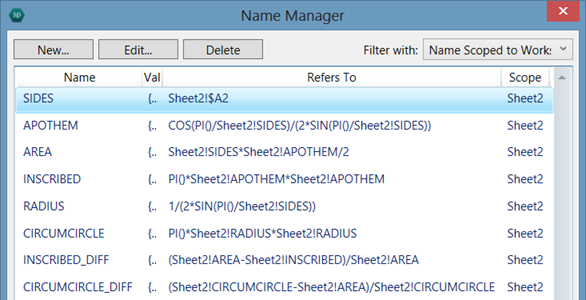Local definitions for names makes it work in Excel

Notice that it is necessary to add each name again, this time as a local name scoped to Sheet2, and it is also necessary to change each formula to explicitly reference the names as local names (e.g., "Sheet2!SIDES" instead of simply "SIDES") in order to make this table work in Excel. Note: this is not an issue with Spread; this is simply how Excel works. You would have similar issues if you created this example in Excel, and would have to use the same workaround to fix it. Using the above definitions for the names referenced in the formulas for the table, instead of the global Workbook definitions, the resulting table will work in Excel now: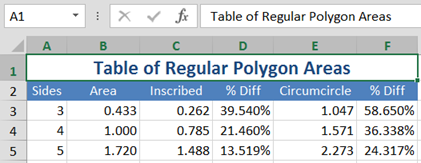Now Polygon Table in Excel 2013 works using local names

Of course, it is perfectly fine to do it without the extra names in Spread, and more efficient since it does not require adding the extra local name definitions. Spread is a powerful tool for analysis, and easier to use than Excel in some cases like this one. The above examples were all created with the Spread Designer for the Spread WPF control, which is shared with the Spread Silverlight control. Neither example requires any coding, but both can be created with code in run time just as easily.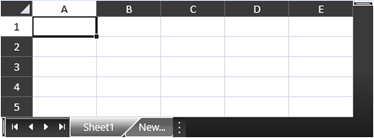Spread WinRT control for Windows 8 Store development

The Spread WinRT control for Windows 8 Store development also features the same Spread Designer tool. The shared SSXML file format enabled using your spreadsheets created in any of the platforms (WPF, Silverlight, or Windows RT) and use them in any of the platforms.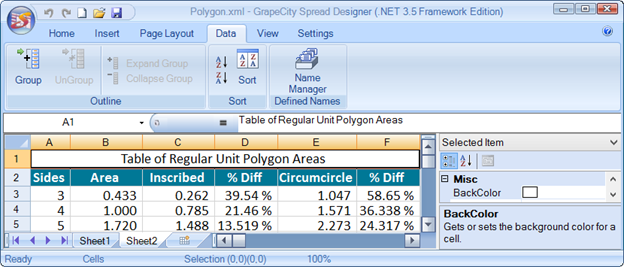Example 2 shown in the upcoming Spread.NET 7 Windows Forms designer

The new version 7 of the Spread.NET controls for Windows Forms and ASP.NET development, coming in April, will feature enhancements including the Name Manager and design-time support for setting and managing names in Spread Designer, and sheet scope names.GrapeCity

GrapeCity Developer Tools